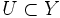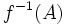# Continuous map

Jump to: navigation, search

## Contents

This article is about a basic definition in topology.
VIEW: Definitions built on this | Facts about this | Survey articles about this
View a complete list of basic definitions in topology

This article defines a property that can be evaluated for a map between topological spaces. Note that the map is not assumed to be continuous

## Definition

### Symbol-free definition

A map from one topological space to another is termed continuous if it satisfies the following equivalent conditions:

### Definition with symbols

Let$X$ and$Y$ be topological spaces. A map$f:X \to Y$ is termed continuous if$f$ satisfies the following equivalent conditions:

•$f^{-1}(U)$ is an open subset of$X$ for every open subset$U \subset Y$
•$f^{-1}(A)$ is a closed subset of$X$ for every closed subset$A \subset Y$

## Facts

### Category

Further information: Category of topological spaces with continuous maps

Continuous maps are the morphisms in the category of topological spaces. In particular, the identity map is continuous, and a composite of continuous maps is also continuous.

For a list of properties that continuous maps may or may not satisfy, refer: